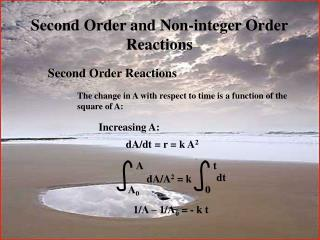# Second Order and Non-integer Order Reactions - PowerPoint PPT PresentationDownload PresentationSecond Order and Non-integer Order Reactions

Second Order and Non-integer Order ReactionsDownload Presentation## Second Order and Non-integer Order Reactions

- - - - - - - - - - - - - - - - - - - - - - - - - - - E N D - - - - - - - - - - - - - - - - - - - - - - - - - - -
##### Presentation Transcript

1. A t dt dA/A2 = k A0 0 Second Order and Non-integer Order Reactions Second Order Reactions The change in A with respect to time is a function of the square of A: Increasing A: dA/dt = r = k A2 1/A – 1/A0 = - k t

2. A t dt dA/A2 = -k A0 0 Decreasing A: 1/A – 1/A0 = k t

3. Increasing A: 1/A – 1/A0 = - k t 1/A = - k t + 1/A0 y = m x + b

4. Example An ozone generator produces ozone based on a reaction that can be modeled based on second order kinetics. If the ozone concentration in a container is 0.0015 g/m3 before the generator is turned on and 0.002 g/m3 after 1 minute, what would the ozone concentration be after 3 minutes? k = 167 m3/g.min

5. 1/A = - k t + 1/A0 1/A = (-167)*3 + (1/0.0015) 1/A = 166 A = .006 g/m3

6. Non-integer Order Reactions dA/dt = r = - k An If A = A0 when t =0, and n is any number not equal to one: (A/A0)1-n –1 = [(n-1) k t]/A0(1-n) k can be found in the same way as in the other cases

7. Half-Life A half life is the amount of time that it takes for ½ of a substance to decay. In other words when t = t1/2, C = 0.5 C0. Zero Order C = C0 – k t t = (C0 –C)/k At half life: t1/2 = (C0 – 0.5 C0)/k = 0.5 C0/k First Order ln(C/C0) = - k t t = ln(C0/C)/k At half life: t1/2 = ln(C0/0.5C0)/k = ln 2/k = 0.693/k

8. By extension: Second Order t1/2 = 1/[k(A0)] Integer Order t1/2 = [((1/2)1-n – 1) – (A0)]/[(n-1)k]

9. Radioactive Decay Radioactive elements disintegrate spontaneously. When this happens high velocity particles are emitted from their nuclei. In natural radioactivity two kinds of particles may be given off, alpha-particles (two neutrons and two protons, a helium nucleus) and beta-particles (high velocity electrons) In addition to these two types of particles natural radioactivity is frequently accompanied by the emission of gamma-rays. A gamma-ray is an electromagnetic wave that is more penetrating than alpha- and beta-particles. They are like x-rays, but have a shorter wavelength.

10. 22688Ra 22286Rn + 42He (alpha-particle) 22286Rn 22286Rn + ( (gamma-ray) Radioactive Decay Example Radium - Ra 22688Ra 226 – mass number – protons + neutrons 88 – atomic number - protons Rn - Radon Radon gas

11. 22688Ra 22286Rn + 42He (alpha-particle) This is the reaction that is occurring when we consider the half-life of Radium The half-life of Radium is 1590 years. In other words, after 1590 years one half of a sample of Radium will be unchanged and one half will be converted to Radon At the end of a second 1590 year period (3180 years) one quarter of the original amount of Radium will remain

12. The decay of Radium is used to provide radiation treatments to some cancer patients. As you can see, it emits radiation which can be hazardous to our health. So it has to be disposed of properly. Since there is not treatment to reduce radioactivity the only thing that can be done with it is to isolate it from the environment. The waste is considered “safe” (radioactivity is near normal background levels) after 99.9% has decayed. How long will this take? If A0 = 1, then A = 0.001 when it is reduced by 99.9% Radioactive decay is a first order reaction, so: t1/2 = 0.693/k 1590 = 0.693/k k = 0.693/1590 = 4.358 x 10-4 years-1

13. We also know that for first order kinetics: ln (A/A0) = -k t Ln (0.001/1) = (-4.358 x 10-4) t t = -6.9078/-4.358 x 10-4 = 15,850 years !

14. k1 k2 A B C More Complex Reactions Consecutive Reactions d[A]/dt = -k1[A] d[B]/dt = k1[A] - k2[B] This system of differential equations can be solved for various initial conditions each of which will give different solutions.

15. k1 k2 A B C This equation is used to model the dissolved oxygen level in a stream. In this case, the oxygen deficit (B) is increased as the result of oxygen being consumed by microorganisms (A B), and the oxygen deficit is decreased by the diffusion of oxygen into the water from the atmosphere (B C) One of the solutions is: [B] = [(k1 A0)/(k2 – k1)] (e-k1 t – e-k2 t) + B0 e-k2 t